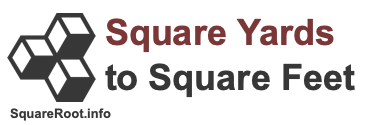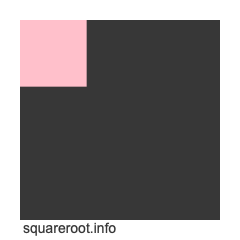3 sq yards to sq feetHere we will explain and show you how to convert 3 square yards to square feet. Before we continue, note that 3 square yards to square feet is also known as 3 sq yards to sq feet, 3 sqyd to sqft, 3 yd2 to ft2, and 3 yd² to ft².

To create a formula to calculate 3 square yards to square feet, we start with the fact that one yard equals three feet. Therefore, this formula is true:

yards × 3 = feet

However, we are dealing with square yards and square feet, which means yards and feet to the 2nd power. Thus, we take both sides of the formula above to the 2nd power to get this result:

yards² × 3² = feet²
yards² × 9 = feet²
yd² × 9 = ft²

Now we know that one square yard is equal to 9 square feet, and the formula to convert square yards to square feet is as follows:

yd² × 9 = ft²

Below is an illustration showing you how one square foot fits into one square yard. The cut out in pink is how one square foot fits into the entire square yard. One square foot is 11.11 percent of a square yard.When we enter 3 square yards into our newly created formula, we get the answer to 3 square yards converted to square feet:

yd² × 9 = ft²
3 × 9
= 27
3 yd² = 27 ft²

Square Yards to Square Feet Converter
Please enter another square yards area in the box below to have it converted to square feet.

Convert  square yards to square feet.

4 sq yards to sq feet
Here is the next area in square yards on our list that we have converted to square feet for you.